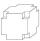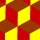# Carpenter

From wooden block carpenter cut off a small cuboid block with half the edge length. How many percent of wood he cut off?

p =  12.5 %

### Step-by-step explanation:

$p=\frac{\left(a\mathrm{/}2\right)\left(b\mathrm{/}2\right)\left(c\mathrm{/}2\right)}{abc}100=\frac{1}{8}\cdot 100=12.5\mathrm{%}$We will be pleased if You send us any improvements to this math problem. Thank you!Tips to related online calculators
Check out our ratio calculator.
Do you want to convert length units?
Tip: Our volume units converter will help you with the conversion of volume units.

## Related math problems and questions:

• Concrete blockDetermine the volume of concrete block whose one edge of the base has a length 3 meters, body diagonal is 13 meters and its height is 12 meters.
• Cube cornersA small cube with an edge length of 2 cm was cut from each corner of a large cube with an edge length of 10 cm. How many cm3 was the body left from the big cube after cutting the small cubes?
• Cuboid enlargementBy how many percent increases the volume of cuboid if its every dimension increases by 30%?
• Volume and body diagonalCalculate how much the volume and body diagonal of the cuboid decrease if we reduce each of its three edges a, b, c by 18%?
• Cuboid edgesCalculate the volume and surface of a cuboid whose edge lengths are in the ratio 2: 3: 4 and the longest edge measures 10cm.
• Reduce of the volumeCalculate how many % reduce the volume of the cube is we reduced length of each edge by 10%.
• Cuboid - ratioFind the volume of a block whose dimensions are in the ratio 2: 3: 4 and the surface is 117 dm2.
• The surface areaHow much percent will the surface area of a 4x5x8 cm block increase if the length of the shortest edge is increased by 2 cm?
• Cuboid wallsThe block's base is a rectangle whose sides have lengths in the ratio of 13: 7. Find the volume of the block in liters if the longer side of the base measures 65 cm, and the height of the block is 1.2 m
• Cuboid - edgesThe cuboid has dimensions in ratio 4: 3: 5, the shortest edge is 12 cm long. Find: (A) the lengths of the remaining edges, (B) the surface of the cuboid, (C) the volume of the cuboid
• The woodenThe wooden block measures 12 cm, 24 cm, and 30 cm. Peter wants to cut it into several identical cubes. At least how many cubes can he get?
• Wood cuboidWhat is the weight of the wood cuboid 15 cm, 20 cm, 3 m if 1 m3 wood weighs 800 kg?
• Ratio of edgesThe dimensions of the cuboid are in a ratio 3: 1: 2. The body diagonal has a length of 28 cm. Find the volume of a cuboid.
• Cuboid and ratioCuboid has dimensions in ratio 1:2:6 and the surface area of the cuboid is 1000 dm2. Calculate the volume of the cuboid.
• Inscribed sphereHow many % of the volume of the cube whose edge is 6 meters long is a volume of a sphere inscribed in that cube?
• Cuboid and ratioFind the dimensions of a cuboid having a volume of 810 cm3 if the lengths of its edges coming from the same vertex are in ratio 2: 3: 5
• The cubeThe cube has an edge of 12 dm. The second cube has an edge exactly 20% longer. How many % is more water in the second cube than in the first cube, if the first cube is full to 3/4 and the second to 3/8?